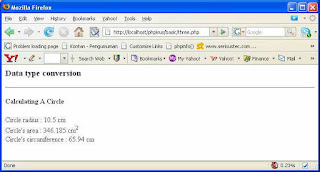### Data type conversion - Meeting 4

Now, we are going to learn on data type's conversion in PHP. Though in PHP we may undeclare the data-type, but we sometimes need to convert the data-type to get the exact results and avoid misleading results. Below is a script which show 3 kinds of how to convert a data-type in PHP. Please read carefully and uncomment one by one of each method of conversions to understand the functions.

<h3>Data type conversion</h3>
<hr><h4>Calculating A Circle</h4>
<?php
\$rad = "10.5 cm";
define("phi",3.14);
//php has 3 ways on converting data types. to try it one by one you can uncomment each command below
/*settype(\$rad,"integer");*/ /*\$rad = intval(\$rad);*/ /*\$rad=(integer)\$rad;*/
/*settype(\$rad,"double");*/ /*\$rad = doubleval(\$rad);*/ /*\$rad=(double)\$rad;*/
/*settype(\$rad,"string);*/ /*\$rad = strval(\$rad);*/ /*\$rad=(string)\$rad;*/
printf("Circle radius : %s <br>",\$rad);
printf("Circle's area : %s cm<sup>2</sup><br>",(\$rad*\$rad*phi));
printf("Circle's circumference : %s cm<br>", (2*phi*\$rad));
?>

The result should be like this:as usual, any questions can be posted via comments and so do the answers. If needed iI will create a new label providing "questions and answers". See you next meeting.